Next: Single-Slit Diffraction Up: Wave Optics Previous: Thin Film Interference

# One-Dimensional Fourier Optics

We have already considered the interference of monochromatic light produced when a plane wave is incident on an opaque screen, coincident with the plane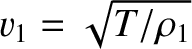, in which a number of narrow (i.e.,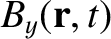, where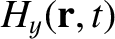is the slit width) slits, running parallel to the-axis, have been cut. Let us now generalize our analysis to take slits of finite width (i.e.,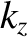) into account. In order to achieve this goal, it is convenient to define the so-called aperture function,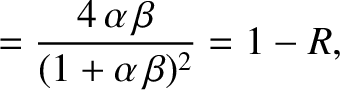, of the screen. This function takes the value zero if the screen is opaque at position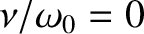, and some constant positive value if it is transparent, and is normalized such that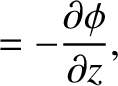. Thus, for the case of a screen with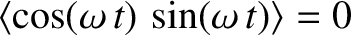identical slits of negligible width, located at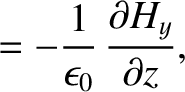, for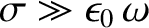, the appropriate aperture function is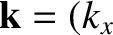(1041)

where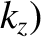is a Dirac delta function.

The wavefunction at the projection screen, generated by the previously mentioned arrangement of slits, when the opaque screen is illuminated by a plane wave of phase angle, wavenumber, and angular frequency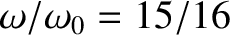, whose direction of propagation subtends an anglewith the-axis, is (see the analysis in Sections 11.2 and 11.4)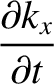(1042)

Here, for the sake of simplicity, we have assumed that the arrangement of slits is symmetric with respect to the plane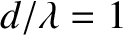, so thatfor any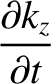. Using the well-known properties of the delta function [see Equation (723)], Equation (1042) can also be written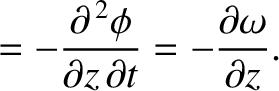(1043)

where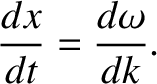(1044)

In the following, we shall assume that Equation (1043) is a general result, and is valid even when the slits in the opaque screen are of finite width (i.e.,). This assumption is equivalent to the assumption that each unblocked section of the screen emits a cylindrical wave in the forward direction that is in phase with the plane wave which illuminates it from behind. The latter assumption is known as Huygen's principle. [Huygen's principle can be justified using advanced electromagnetic theory (Jackson 1975), but such a proof lies well beyond the scope of this book.] The interference/diffraction function,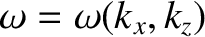, is the Fourier transform of the aperture function,. This is an extremely powerful result. It implies that we can calculate the far-field interference/diffraction pattern associated with any arrangement of parallel slits, of arbitrary width, by Fourier transforming the associated aperture function. Once we have determined the interference/diffraction function,, the intensity of the interference/diffraction pattern appearing on the projection screen is readily obtained from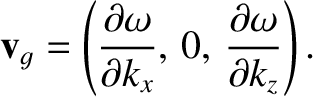(1045)Next: Single-Slit Diffraction Up: Wave Optics Previous: Thin Film Interference
Richard Fitzpatrick 2013-04-08